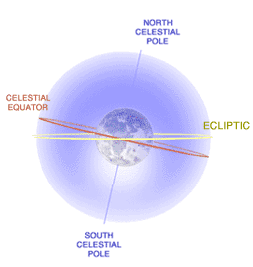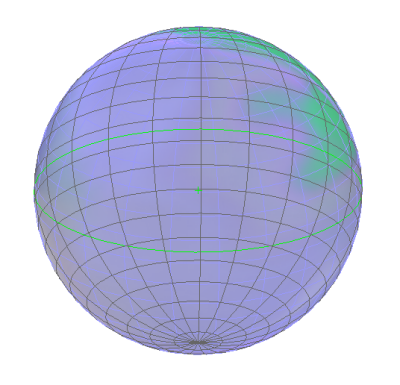# Numb3rs 2x03: Obsession

In the episode Obsession, a photographer is killed while attempting to take tabloid sex photos. In an effort to retrace the photographers steps, the FBI examine his camera. They find a series of photos of a basketball hoop, each showing the hoop's shadow. Since each photograph is timestamped, Charlie was able to use the behavior of the hoop's shadow to pinpoint the longitude and latitude where the photos had been taken.

In order to make calculations involving the position of the sun in the sky, Charlie used a technique called spherical astronomy. In this subject, all observable planetary bodies and stars are assumed to lie on the celestial sphere, an imaginary rotating sphere of gigantic radius concentric to the Earth. In ancient Greece, this model was believed to be an accurate representation of the universe. Although we now know that these observed rotations are actually caused by the rotation of the earth itself rather than some super-sphere, scientists still use the techniques of spherical astronomy, which are often quite accurate and simplify calculations a great deal.

Spherical astronomy works particularly well for calculations involving constellations and other far away objects, as their movement is barely perceptible from Earth (the movement of stationary bodies will be solely determined by the rotation of earth). When doing long term calculations with closer bodies, however, other considerations must be taken into account. For example, the position of the Sun on the celestial sphere (i.e. in relation to all the other stars) is constantly shifting, moving a little under one degree eastward in each 24 hour period. The sun also moves due to the tilting of the earth on it's axis. The path traced by the sun on the celestial sphere is called the ecliptic.The tilt of the Earth on its axis and the eccentricity of its orbit around the sun results in more complex solar movements than described here. For more information, (and some great pictures) see this article on the analemma , a figure eight path the sun traces in the sky in the course of a year.
The Celestial Sphere:
1. How long will it take for the Sun to return to its original position in the celestial sphere?
2. The north star is famous for being virtually stationary in the night sky. From the perspective of the celestial sphere, how is Polaris accomplishing this feat?
3. Assume for the sake of simplicity that the sun remains fixed on the celestial sphere during a 24 hour time period, how would you describe the set of points on Earth that experience the Sun directly overhead at some time during this period?

The assumption of a celestial sphere makes it relatively easy to describe the position of a celestial body from the perspective of a person standing on the earth. The position of the sun, for example, can be related by two angles: the altitude is the angle between the sun and the observable horizon, whereas the the azimuth is the angle on the horizon measured clockwise from due south. From an observer on the earth's prospective, all points with the same altitude form a circle in the sky at that altitude, whereas all points with the same azimuth form a semicircle in the sky, passing directly overhead and intersecting the horizon at a right angles.

1. When a tall pole casts a shadow, what determines the length of the shadow? What determines it's position?
2. How would a shadow cast at at 3pm compare to a shadow cast at 3:30?
3. Two basketball hoops (each 10 feet tall) are photographed at precisely the same afternoon time. Assume that these basketball hoops are at the same latitude, but one is 200 miles east of the other. How could you differentiate the photographs?
4. Assume a basketball hoop is casting a 5 foot shadow. What is the sun's altitude at the time?The method of representing points on a sphere that we have used here can be extended to spherical coordinates. You are no doubt accustomed to discussing points in 2-dimensional space, or the Euclidean plane as pairs of points (x,y). This idea can be extended by adding a third z-axis. Imagine that the xy-plane that you are used to lies on a chalkboard, the z axis will be coming directly out of the chalkboard, perpendicular to both the x and y axes. Now we can describe all points in three dimensional space as (x,y,z).

Spherical coordinates provide a different, and often useful way of describing the position of objects in three dimensional space. The basic principle of this coordinate system is that every point lies on some sphere centered at the origin, so one need only specify which sphere (using radius), and the precise coordinates of the point on said sphere (the angles of azimuth and altitude). Therefore a point is written in spherical coordinates as (r, θ, φ) where θ represents the azimuth, φ represents altitude, and r represents the distance between the point and the origin.

Spherical Coordinates
1. Describe the set of all points in three space with a radius of 5.
2. Describe the set of all points in three space with an altitude of 45°.
3. Describe the set of points in three space with an azimuth of 90°.
4. Challenge: Come up with a formula for converting the spherical coordinates (r,θ, φ) of a point into in Cartesian coordinates. (x,y,z)
Note: You will need the distance formula in three dimensions, and more than one right triangle.